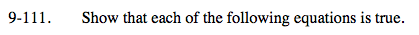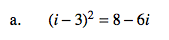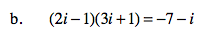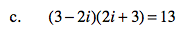### Home > A2C > Chapter 9 > Lesson 9.2.3 > Problem9-111

9-111.
1. Show that each of the following equations is true. Homework Help ✎

1. (i − 3)2 = 8 − 6i

2. (2i − 1)(3i + 1) = −7 − i

3. (3 − 2i)(2i + 3) = 13Start by multiplying.
i 2 − 6i + 9 = 8 − 6i

i 2 = −1, so i 2 − 6i + 9 = −1 − 6i + 9 = 8 − 6iSee part (a).See part (a).